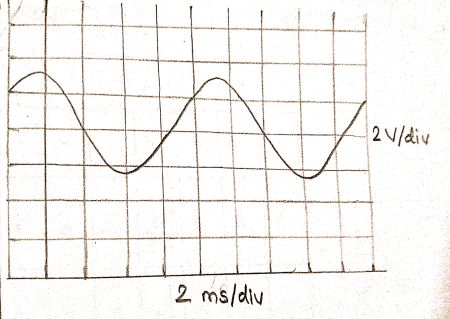# Using the picture of the signal of voltage versus time from the screen of the oscilloscope and...

## Question:

Using the picture of the signal of voltage versus time from the screen of the oscilloscope and assuming that the settings on the oscilloscope are 2 V/div and 2 ms/div, calculate the following:

a) Amplitude (express as a whole number)

b) Period (in milliseconds)

c) Frequency (round off to the tens place)

d) {eq}V_{rms}{/eq} value (express as a whole number)

e) {eq}V_{PP}{/eq} (peak to peak) value (express as a whole number)## Amplitude:

Amplitude of a wave is maximum displacement of particle from it's mean position.

Usually amplitude is represented with letter A.

Frequency:

Number of oscillation completed per unit time ( 1 1second) is called frequecy.

IT is represented with letter {eq}\nu {/eq}.

SI unit of frequency is Hz.

Become a Study.com member to unlock this answer! Create your account

Number of division displaced from mean position {eq}= 1.5 {/eq} div

_Part(A)

Amplitiude {eq}A = 2 \ \ V\div \times 1.5 \ \ div {/eq}

{eq}A = 3 \...